# NCERT Exemplar Solutions for Class 11 Maths Chapter 7 Permutations and Combinations

NCERT Exemplar Solutions for Class 11 Maths Chapter 7 Permutations and Combinations are an educational aid for students that help them solve and learn simple and difficult tasks. It includes a complete set of questions organized with an advanced level of difficulty, which provide students ample opportunity to apply combination and skills. Get free NCERT Exemplar Solutions for Class 11 Maths, Chapter 7 Permutations and Combinations. NCERT Exemplar Solutions will help the students to understand the concept of Permutation and combinations, properties, and some important formulae. These solutions can, not only help students to clear their doubts but also to prepare more efficiently.

The 7th Chapter, Permutations and Combinations of NCERT Exemplar Solutions for Class 11 Maths explains the permutation of ‘n’ different objects. The main aim of these solutions formulated by BYJU’S experts is to provide fundamental aspects of Maths, which in turn, helps students to understand every concept clearly. Permutations and Combinations are some of the major ways in which objects can be selected from a set. The sets, which ultimately form subsets, selected cannot usually be replaced. A permutation can be mentioned when the order of things matters whereas, a combination is simply the order of things that do not matter.

Permutations are of two types:

1. Permutations without Repetition
2. Permutations with Repetition

Combinations are also of two types:

1. Combinations without Repetition
2. Combinations with Repetition

In Chapter 7, students will learn to solve exemplar problems based on the topics given below;

• Fundamental Principle of Counting
• Multiplication principle
• Permutations when all the objects are distinct
• Factorial Notation
• Permutations when all the objects are not distinct objects
• Problems based on combinations

## Download PDF Of NCERT Exemplar Solutions for Class 11 Maths Chapter 7 Permutations and Combinations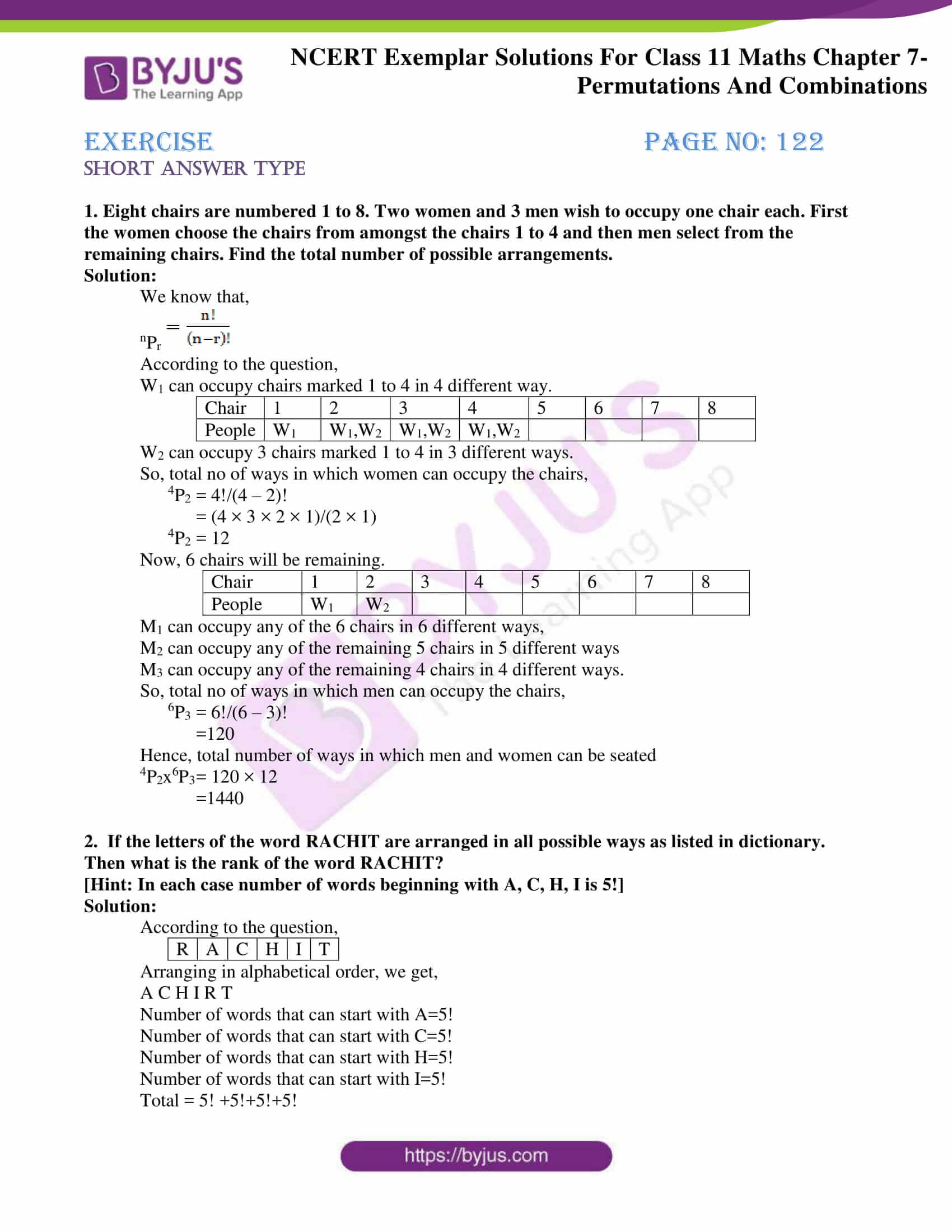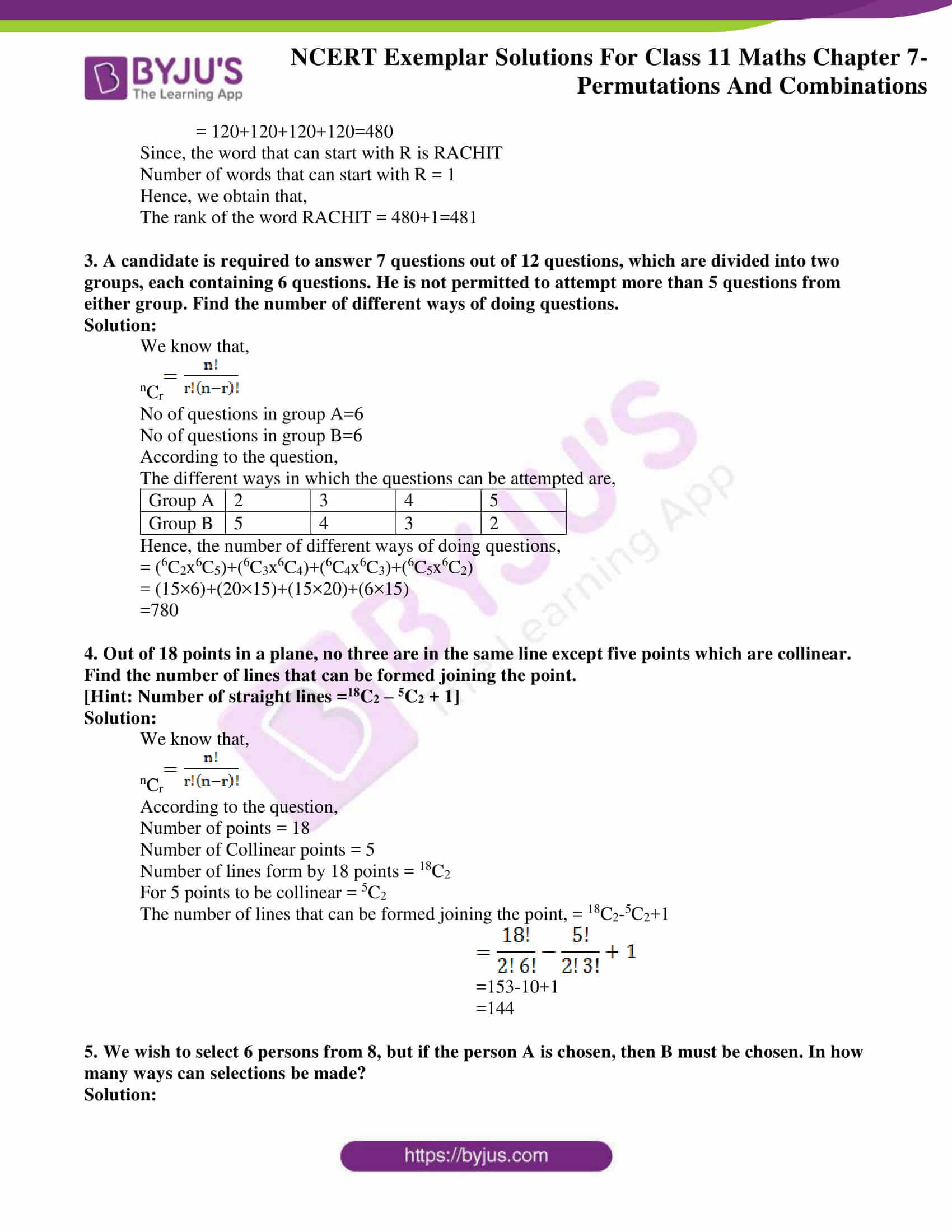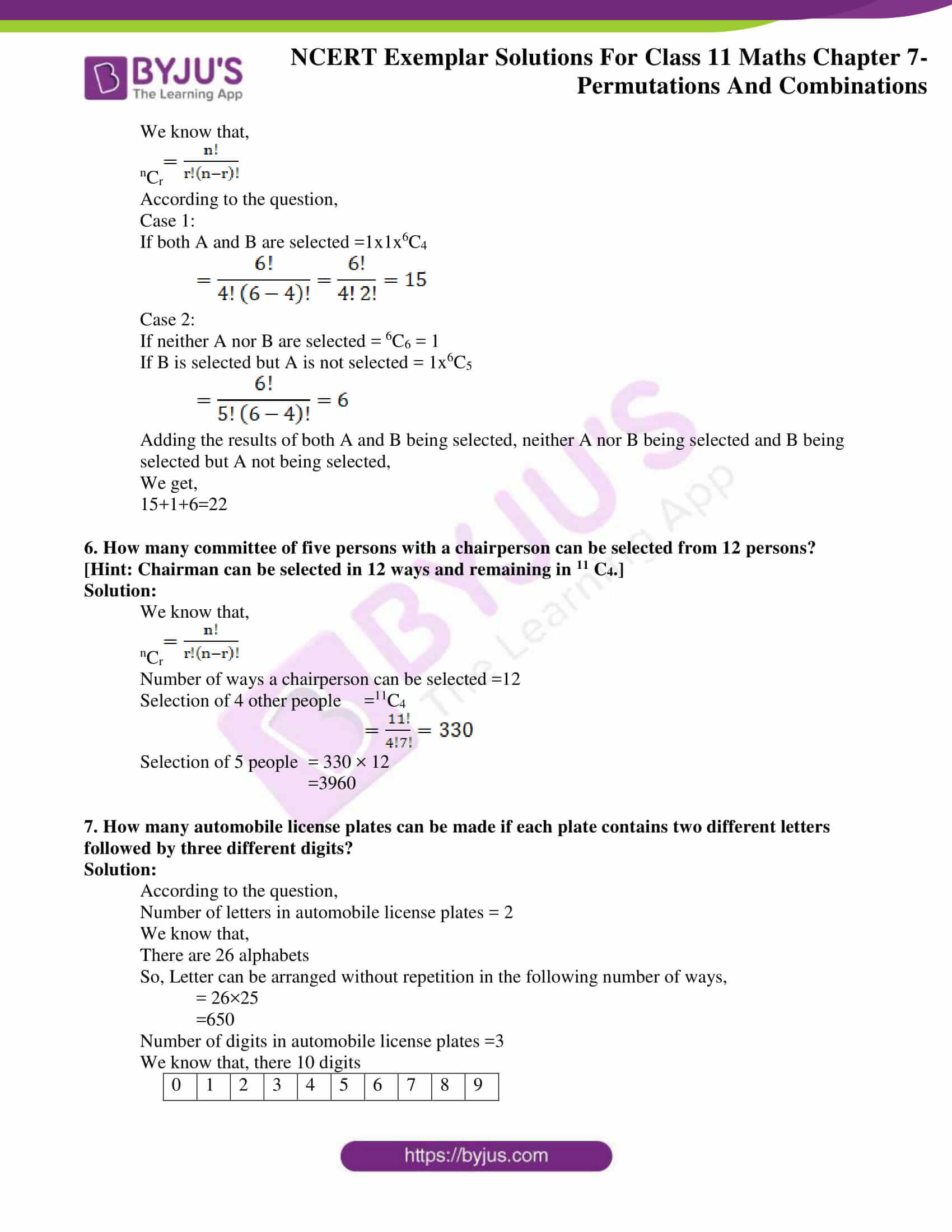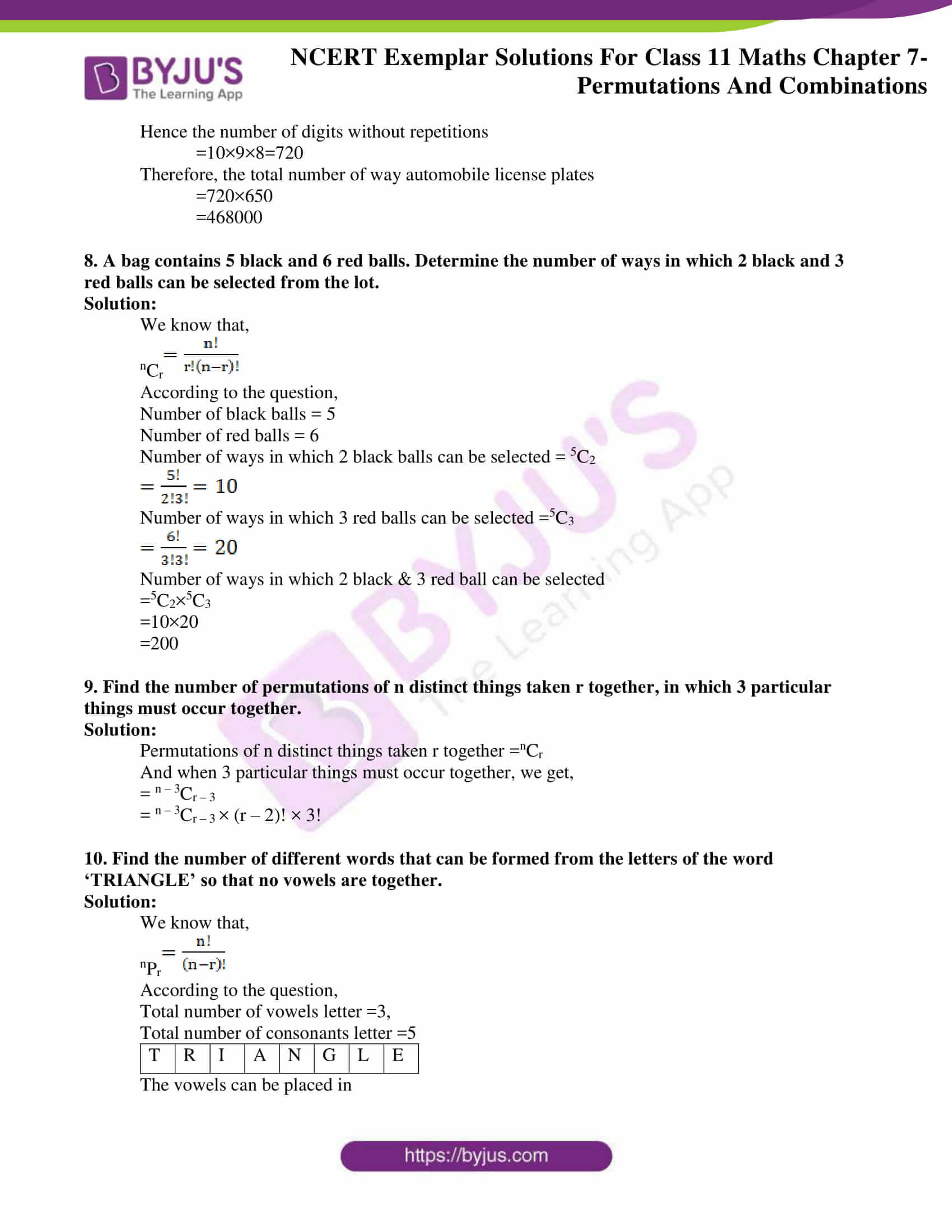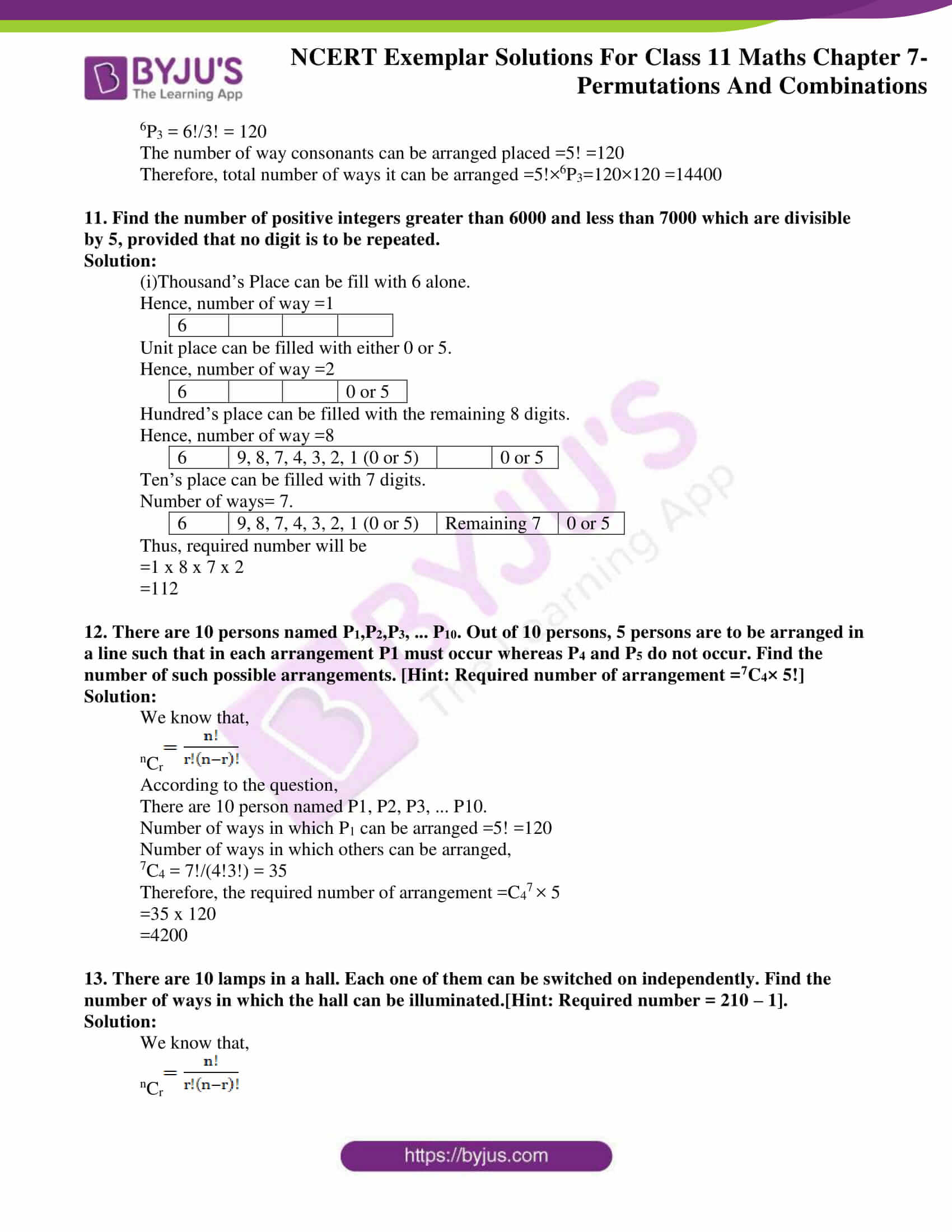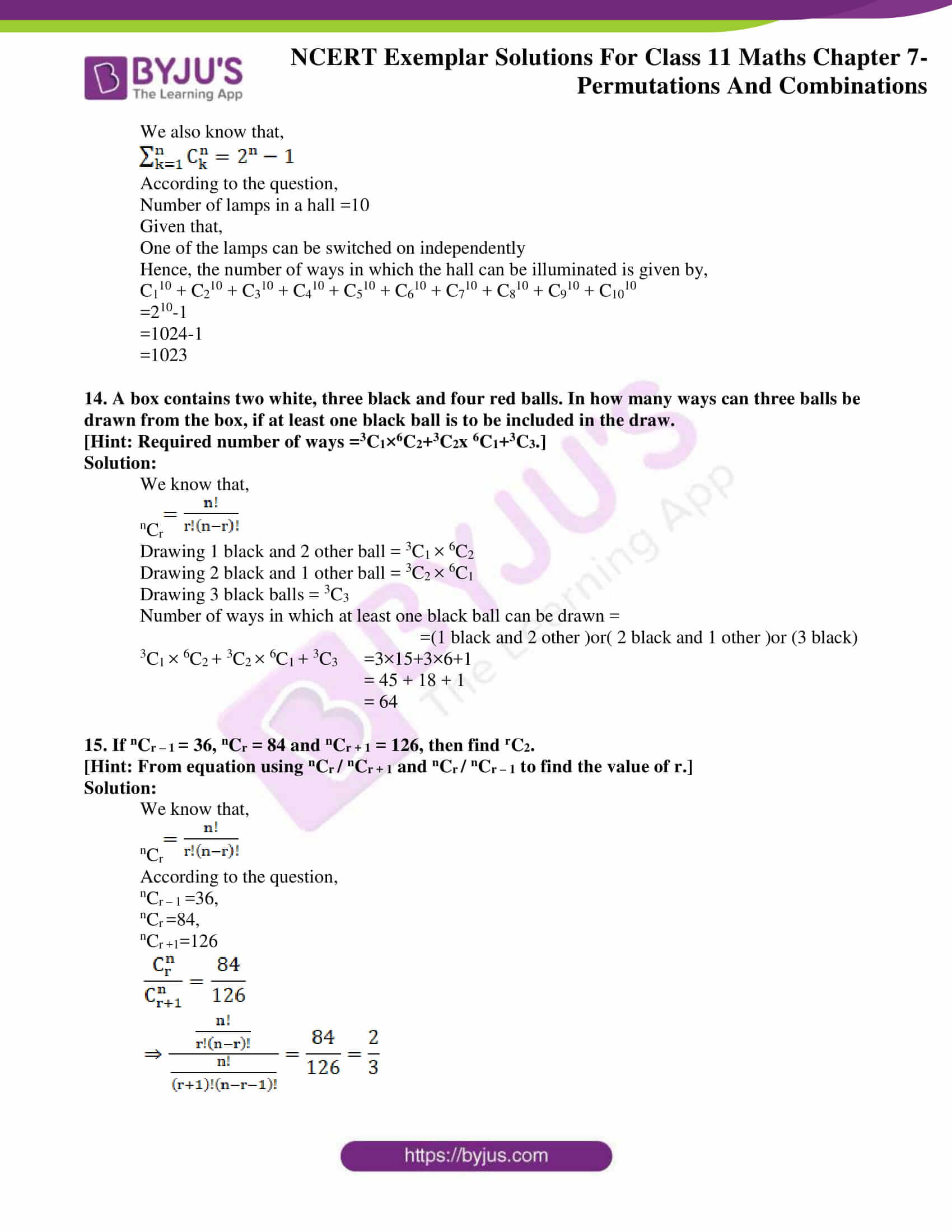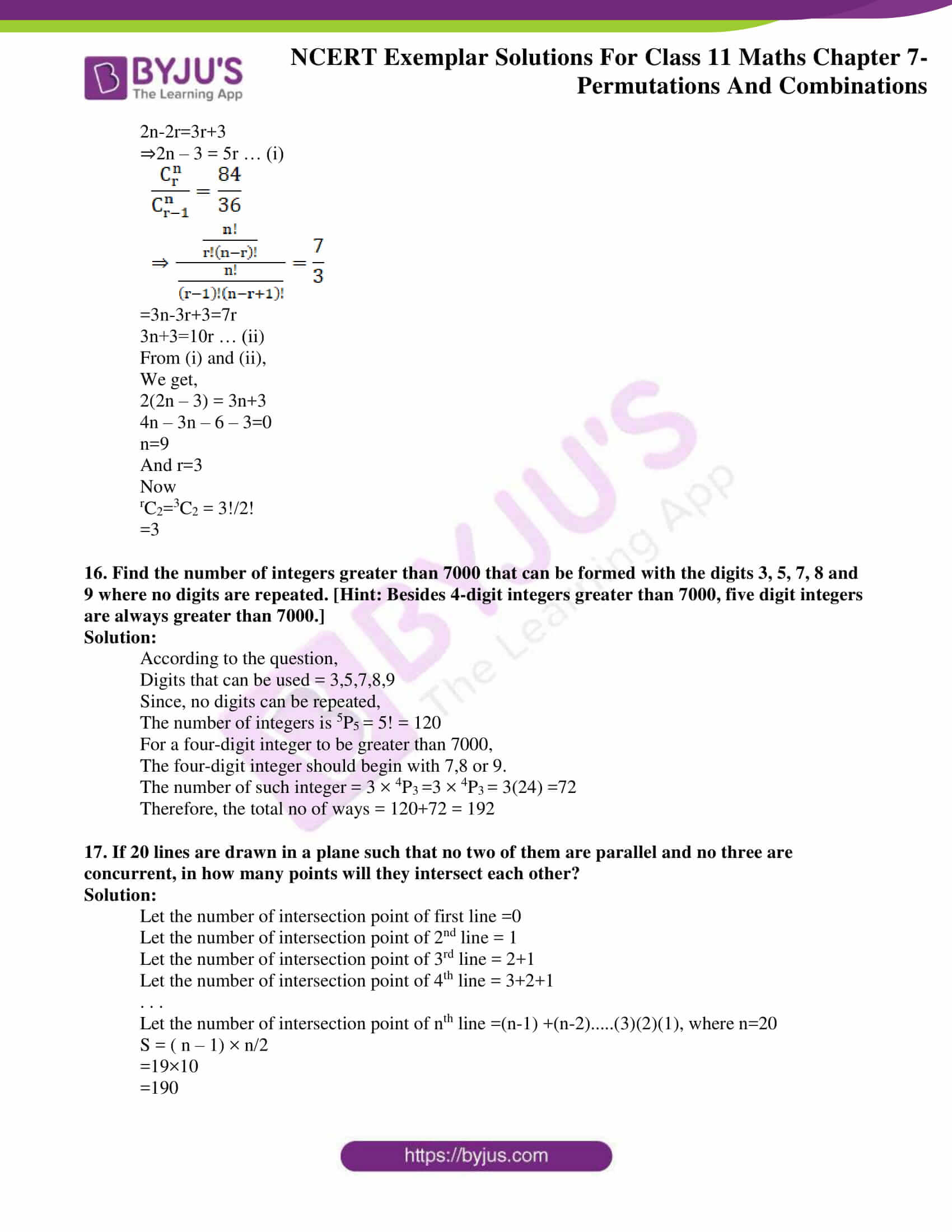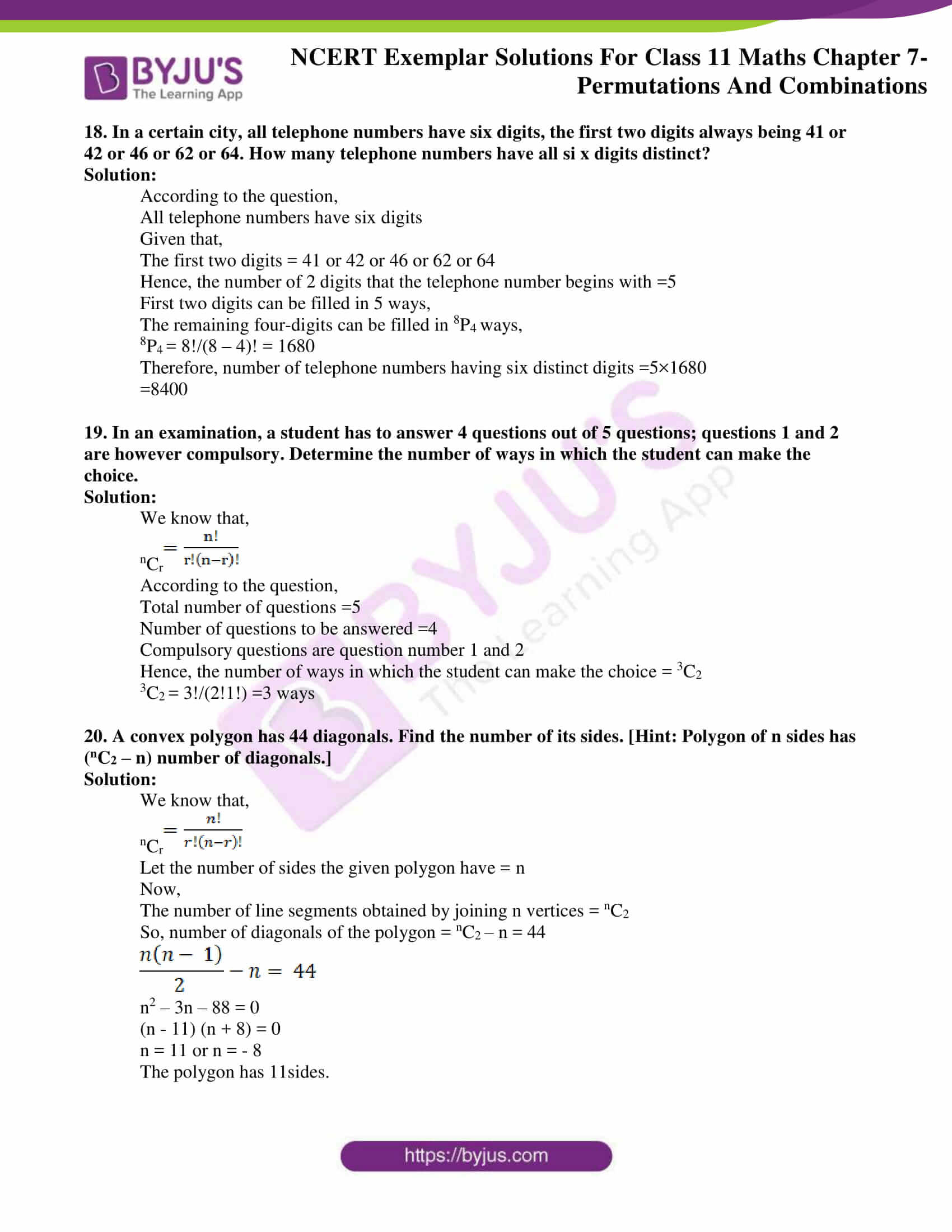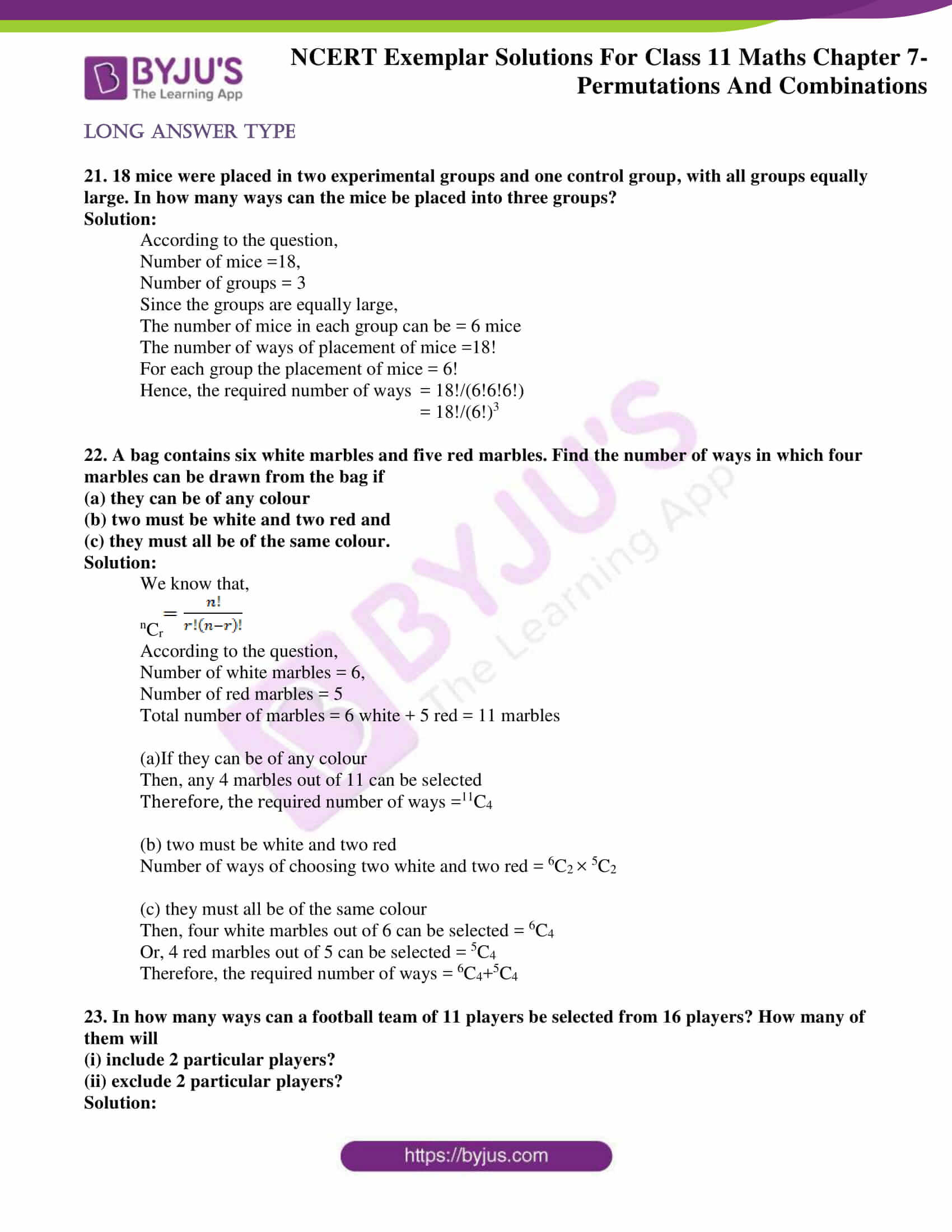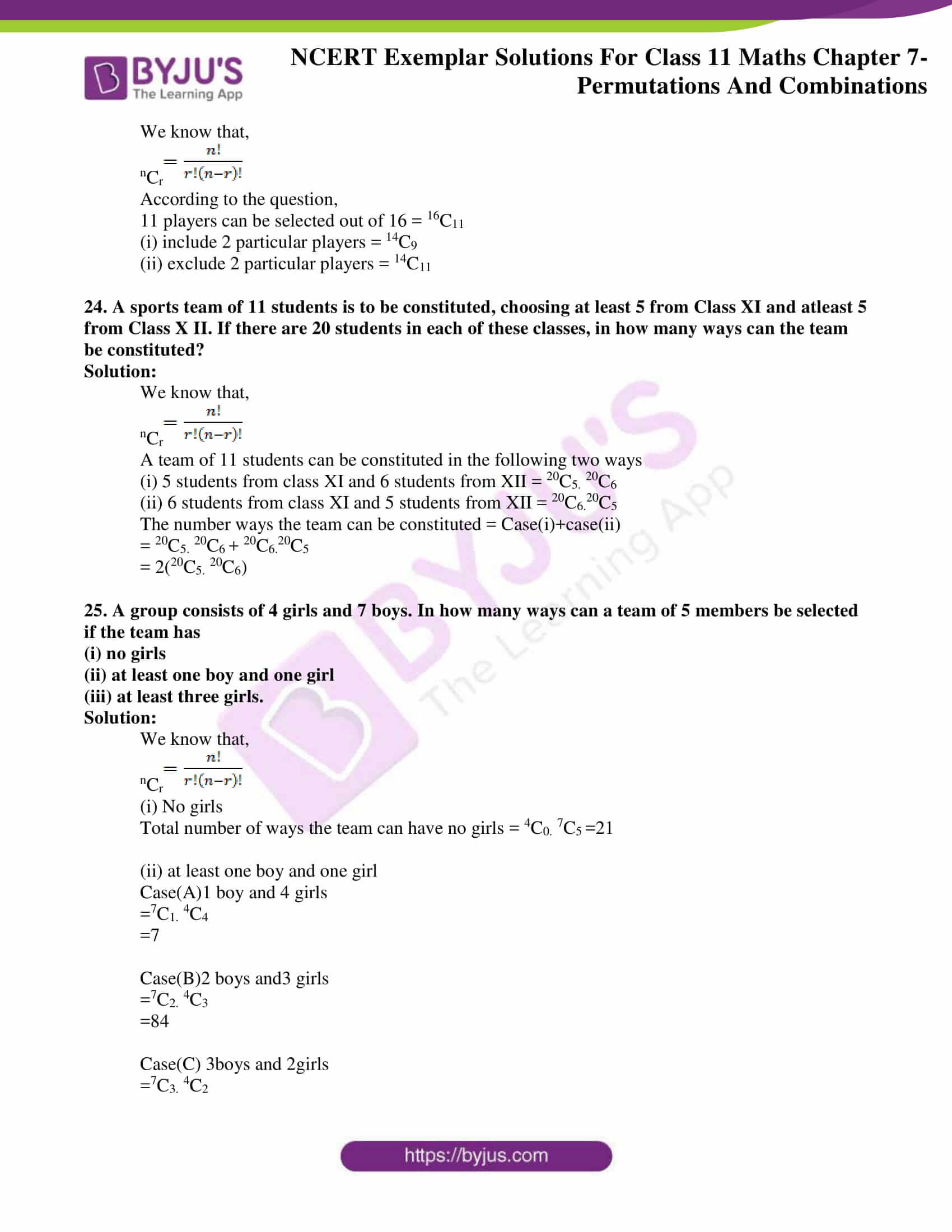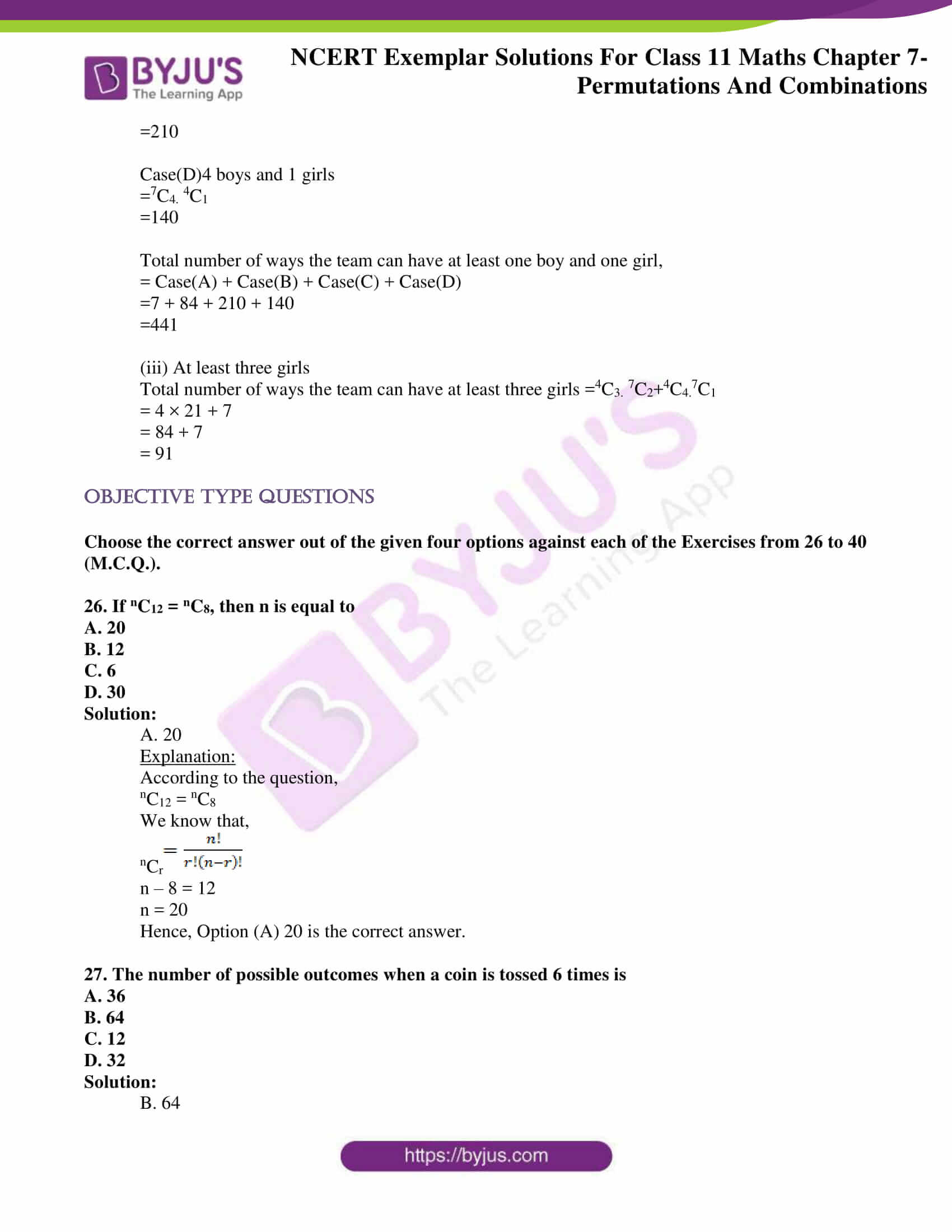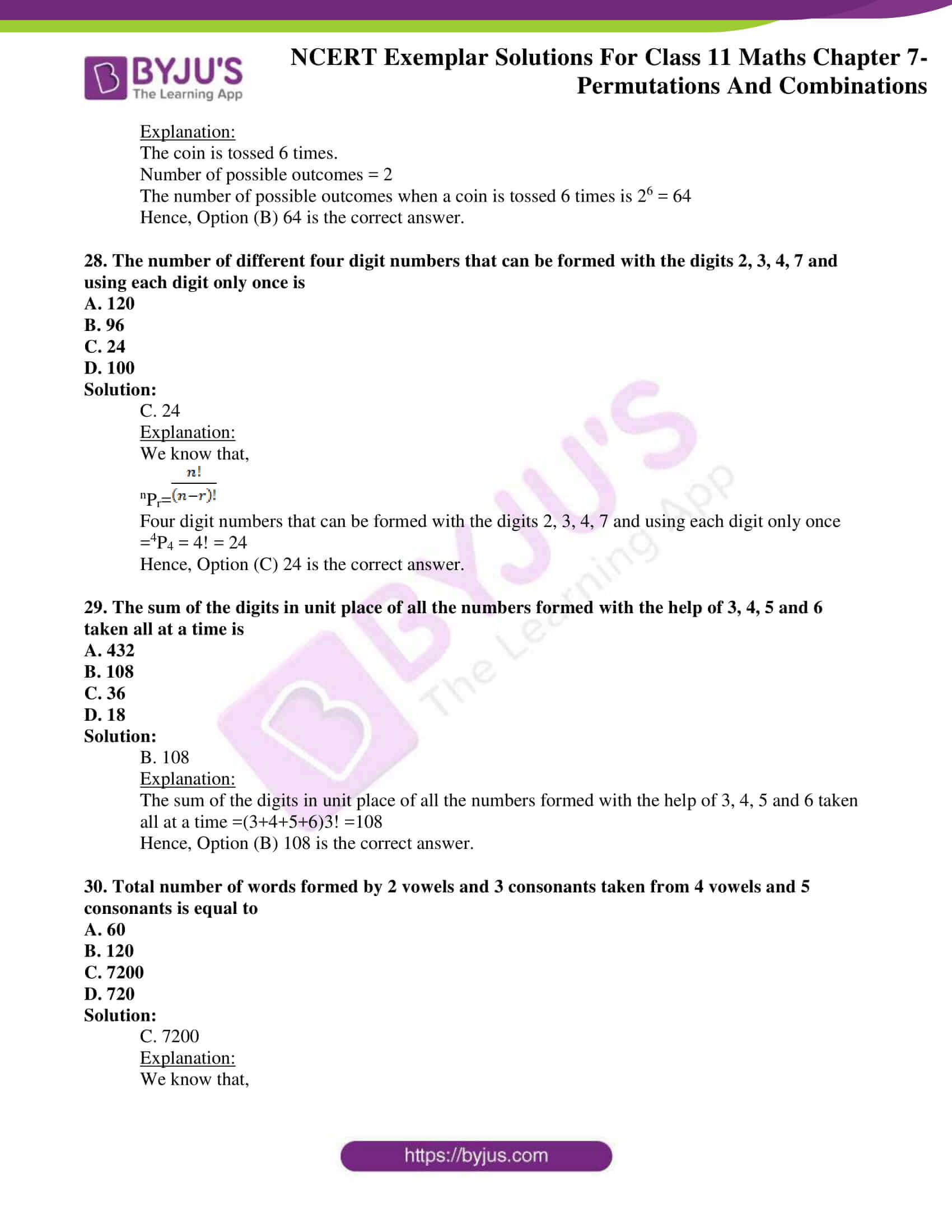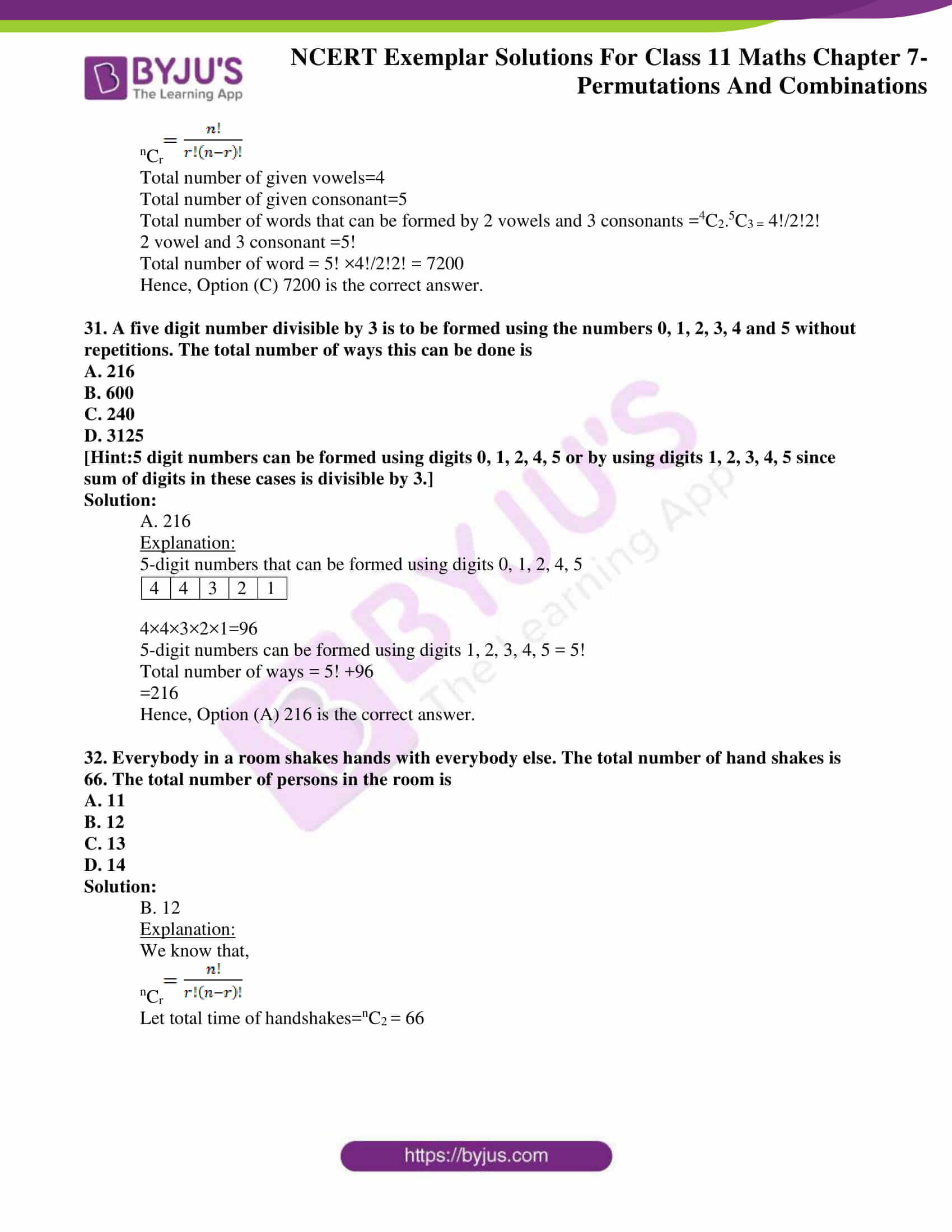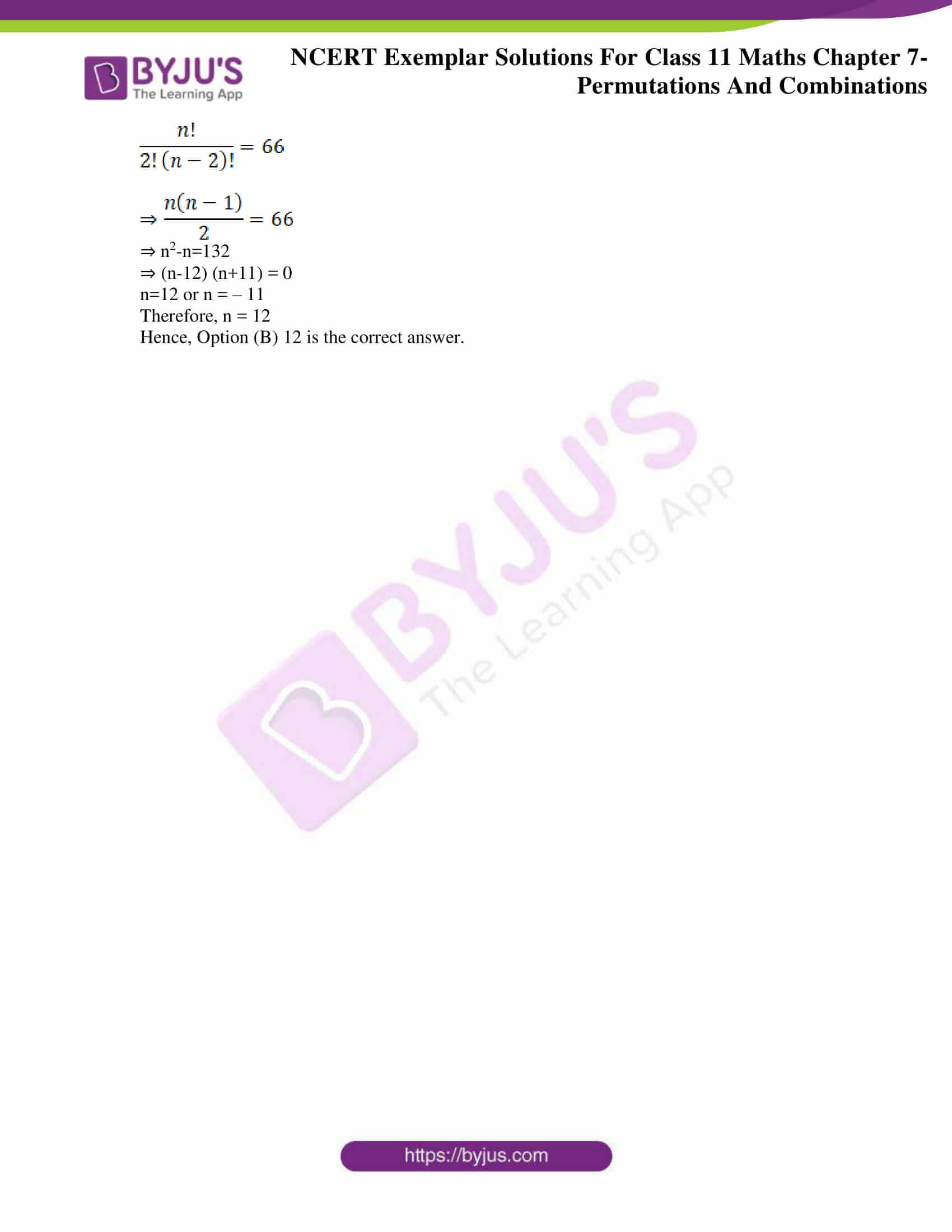### Access Answers to NCERT Exemplar Class 11 Maths Solutions Chapter 7 Permutations and Combinations

Exercise Page No: 122

1. Eight chairs are numbered 1 to 8. Two women and 3 men wish to occupy one chair each. First the women choose the chairs from amongst the chairs 1 to 4 and then men select from the remaining chairs. Find the total number of possible arrangements.

Solution:

We know that,

nPr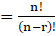According to the question,

W1 can occupy chairs marked 1 to 4 in 4 different way.

 Chair 1 2 3 4 5 6 7 8 People W1 W1,W2 W1,W2 W1,W2

W2 can occupy 3 chairs marked 1 to 4 in 3 different ways.

So, total no of ways in which women can occupy the chairs,

4P2 = 4!/(4 – 2)!

= (4 × 3 × 2 × 1)/(2 × 1)

4P2 = 12

Now, 6 chairs will be remaining.

 Chair 1 2 3 4 5 6 7 8 People W1 W2

M1 can occupy any of the 6 chairs in 6 different ways,

M2 can occupy any of the remaining 5 chairs in 5 different ways

M3 can occupy any of the remaining 4 chairs in 4 different ways.

So, total no of ways in which men can occupy the chairs,

6P3 = 6!/(6 – 3)!

=120

Hence, total number of ways in which men and women can be seated

4P2x6P3 = 120 × 12

=1440

2. If the letters of the word RACHIT are arranged in all possible ways as listed in dictionary. Then what is the rank of the word RACHIT?
[Hint: In each case number of words beginning with A, C, H, I is 5!]

Solution:

According to the question,

 R A C H I T

Arranging in alphabetical order, we get,

A C H I R T

Total = 5! +5!+5!+5!

= 120+120+120+120=480

Hence, we obtain that,

The rank of the word RACHIT = 480+1=481

3. A candidate is required to answer 7 questions out of 12 questions, which are divided into two groups, each containing 6 questions. He is not permitted to attempt more than 5 questions from either group. Find the number of different ways of doing questions.

Solution:

We know that,

nCr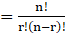No of questions in group A=6

No of questions in group B=6

According to the question,

The different ways in which the questions can be attempted are,

 Group A 2 3 4 5 Group B 5 4 3 2

Hence, the number of different ways of doing questions,

= (6C2x6C5)+(6C3x6C4)+(6C4x6C3)+(6C5x6C2)

= (15×6)+(20×15)+(15×20)+(6×15)

=780

4. Out of 18 points in a plane, no three are in the same line except five points which are collinear. Find the number of lines that can be formed joining the point.
[Hint: Number of straight lines =18C2 – 5C2 + 1]

Solution:

We know that,

nCrAccording to the question,

Number of points = 18

Number of Collinear points = 5

Number of lines form by 18 points = 18C2

For 5 points to be collinear = 5C2

The number of lines that can be formed joining the point, = 18C25C2+1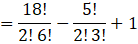=153-10+1

=144

5. We wish to select 6 persons from 8, but if the person A is chosen, then B must be chosen. In how many ways can selections be made?

Solution:

We know that,

nCrAccording to the question,

Case 1:

If both A and B are selected =1x1x6C4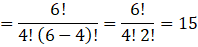Case 2:

If neither A nor B are selected = 6C6 = 1

If B is selected but A is not selected = 1x6C5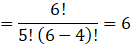Adding the results of both A and B being selected, neither A nor B being selected and B being selected but A not being selected,

We get,

15+1+6=22

6. How many committee of five persons with a chairperson can be selected from 12 persons? [Hint: Chairman can be selected in 12 ways and remaining in 11 C4.]

Solution:

We know that,

nCrNumber of ways a chairperson can be selected =12

Selection of 4 other people =11C4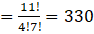Selection of 5 people = 330 × 12

=3960

7. How many automobile license plates can be made if each plate contains two different letters followed by three different digits?

Solution:

According to the question,

Number of letters in automobile license plates = 2

We know that,

There are 26 alphabets

So, Letter can be arranged without repetition in the following number of ways,

= 26×25

=650

Number of digits in automobile license plates =3

We know that, there 10 digits

 0 1 2 3 4 5 6 7 8 9

Hence the number of digits without repetitions

=10×9×8=720

Therefore, the total number of way automobile license plates

=720×650

=468000

8. A bag contains 5 black and 6 red balls. Determine the number of ways in which 2 black and 3 red balls can be selected from the lot.

Solution:

We know that,

nCrAccording to the question,

Number of black balls = 5

Number of red balls = 6

Number of ways in which 2 black balls can be selected = 5C2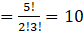Number of ways in which 3 red balls can be selected =5C3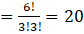Number of ways in which 2 black & 3 red ball can be selected

=5C2×5C3

=10×20

=200

9. Find the number of permutations of n distinct things taken r together, in which 3 particular things must occur together.

Solution:

Permutations of n distinct things taken r together =nCr

And when 3 particular things must occur together, we get,

n – 3Cr – 3

n – 3Cr – 3 × (r – 2)! × 3!

10. Find the number of different words that can be formed from the letters of the word ‘TRIANGLE’ so that no vowels are together.

Solution:

We know that,

nPrAccording to the question,

Total number of vowels letter =3,

Total number of consonants letter =5

 T R I A N G L E

The vowels can be placed in

6P3 = 6!/3! = 120

The number of way consonants can be arranged placed =5! =120

Therefore, total number of ways it can be arranged =5!×6P3=120×120 =14400

11. Find the number of positive integers greater than 6000 and less than 7000 which are divisible by 5, provided that no digit is to be repeated.

Solution:

(i)Thousand’s Place can be fill with 6 alone.

Hence, number of way =1

 6

Unit place can be filled with either 0 or 5.

Hence, number of way =2

 6 0 or 5

Hundred’s place can be filled with the remaining 8 digits.

Hence, number of way =8

 6 9, 8, 7, 4, 3, 2, 1 (0 or 5) 0 or 5

Ten’s place can be filled with 7 digits.

Number of ways= 7.

 6 9, 8, 7, 4, 3, 2, 1 (0 or 5) Remaining 7 0 or 5

Thus, required number will be

=1 x 8 x 7 x 2

=112

12. There are 10 persons named P1,P2,P3, … P10. Out of 10 persons, 5 persons are to be arranged in a line such that in each arrangement P1 must occur whereas P4 and P5 do not occur. Find the number of such possible arrangements. [Hint: Required number of arrangement =7C4× 5!]

Solution:

We know that,

nCrAccording to the question,

There are 10 person named P1, P2, P3, … P10.

Number of ways in which P1 can be arranged =5! =120

Number of ways in which others can be arranged,

7C4 = 7!/(4!3!) = 35

Therefore, the required number of arrangement =C47 × 5

=35 x 120

=4200

13. There are 10 lamps in a hall. Each one of them can be switched on independently. Find the number of ways in which the hall can be illuminated.[Hint: Required number = 210 – 1].

Solution:

We know that,

nCrWe also know that,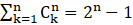According to the question,

Number of lamps in a hall =10

Given that,

One of the lamps can be switched on independently

Hence, the number of ways in which the hall can be illuminated is given by,

C110 + C210 + C310 + C410 + C510 + C610 + C710 + C810 + C910 + C1010

=210-1

=1024-1

=1023

14. A box contains two white, three black and four red balls. In how many ways can three balls be drawn from the box, if at least one black ball is to be included in the draw.
[Hint: Required number of ways =3C1×6C2+3C26C1+3C3.]

Solution:

We know that,

nCrDrawing 1 black and 2 other ball = 3C1 × 6C2

Drawing 2 black and 1 other ball = 3C2 × 6C1

Drawing 3 black balls = 3C3

Number of ways in which at least one black ball can be drawn =

=(1 black and 2 other )or( 2 black and 1 other )or (3 black)

3C1 × 6C2 + 3C2 × 6C1 + 3C3 =3×15+3×6+1

= 45 + 18 + 1

= 64

15. If nCr – 1 = 36, nCr = 84 and nCr + 1 = 126, then find rC2.

[Hint: From equation using nCr / nCr + 1 and nCr / nCr – 1 to find the value of r.]

Solution:

We know that,

nCrAccording to the question,

nCr – 1 =36,

nCr =84,

nCr +1=126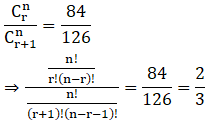2n-2r=3r+3

⇒2n – 3 = 5r … (i)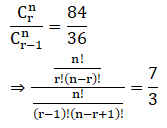=3n-3r+3=7r

3n+3=10r … (ii)

From (i) and (ii),

We get,

2(2n – 3) = 3n+3

4n – 3n – 6 – 3=0

n=9

And r=3

Now

rC2=3C2 = 3!/2!

=3

16. Find the number of integers greater than 7000 that can be formed with the digits 3, 5, 7, 8 and 9 where no digits are repeated. [Hint: Besides 4-digit integers greater than 7000, five digit integers are always greater than 7000.]

Solution:

According to the question,

Digits that can be used = 3,5,7,8,9

Since, no digits can be repeated,

The number of integers is 5P5 = 5! = 120

For a four-digit integer to be greater than 7000,

The four-digit integer should begin with 7,8 or 9.

The number of such integer = 3 × 4P3 =3 × 4P3 = 3(24) =72

Therefore, the total no of ways = 120+72 = 192

17. If 20 lines are drawn in a plane such that no two of them are parallel and no three are concurrent, in how many points will they intersect each other?

Solution:

Let the number of intersection point of first line =0

Let the number of intersection point of 2nd line = 1

Let the number of intersection point of 3rd line = 2+1

Let the number of intersection point of 4th line = 3+2+1

. . .

Let the number of intersection point of nth line =(n-1) +(n-2)…..(3)(2)(1), where n=20

S = ( n – 1) × n/2

=19×10

=190

18. In a certain city, all telephone numbers have six digits, the first two digits always being 41 or 42 or 46 or 62 or 64. How many telephone numbers have all si x digits distinct?

Solution:

According to the question,

All telephone numbers have six digits

Given that,

The first two digits = 41 or 42 or 46 or 62 or 64

Hence, the number of 2 digits that the telephone number begins with =5

First two digits can be filled in 5 ways,

The remaining four-digits can be filled in 8P4 ways,

8P4 = 8!/(8 – 4)! = 1680

Therefore, number of telephone numbers having six distinct digits =5×1680

=8400

19. In an examination, a student has to answer 4 questions out of 5 questions; questions 1 and 2 are however compulsory. Determine the number of ways in which the student can make the choice.

Solution:

We know that,

nCrAccording to the question,

Total number of questions =5

Number of questions to be answered =4

Compulsory questions are question number 1 and 2

Hence, the number of ways in which the student can make the choice = 3C2

3C2 = 3!/(2!1!) =3 ways

20. A convex polygon has 44 diagonals. Find the number of its sides. [Hint: Polygon of n sides has (nC2 – n) number of diagonals.]

Solution:

We know that,

nCr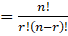Let the number of sides the given polygon have = n

Now,

The number of line segments obtained by joining n vertices = nC2

So, number of diagonals of the polygon = nC2 – n = 44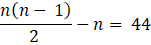n2 – 3n – 88 = 0

(n – 11) (n + 8) = 0

n = 11 or n = – 8

The polygon has 11sides.

21. 18 mice were placed in two experimental groups and one control group, with all groups equally large. In how many ways can the mice be placed into three groups?

Solution:

According to the question,

Number of mice =18,

Number of groups = 3

Since the groups are equally large,

The number of mice in each group can be = 6 mice

The number of ways of placement of mice =18!

For each group the placement of mice = 6!

Hence, the required number of ways = 18!/(6!6!6!)

= 18!/(6!)3

22. A bag contains six white marbles and five red marbles. Find the number of ways in which four marbles can be drawn from the bag if
(a) they can be of any colour
(b) two must be white and two red and
(c) they must all be of the same colour.

Solution:

We know that,

nCrAccording to the question,

Number of white marbles = 6,

Number of red marbles = 5

Total number of marbles = 6 white + 5 red = 11 marbles

(a)If they can be of any colour

Then, any 4 marbles out of 11 can be selected

Therefore, the required number of ways =11C4

(b) two must be white and two red

Number of ways of choosing two white and two red = 6C2 × 5C2

(c) they must all be of the same colour

Then, four white marbles out of 6 can be selected = 6C4

Or, 4 red marbles out of 5 can be selected = 5C4

Therefore, the required number of ways = 6C4+5C4

23. In how many ways can a football team of 11 players be selected from 16 players? How many of them will
(i) include 2 particular players?
(ii) exclude 2 particular players?

Solution:

We know that,

nCrAccording to the question,

11 players can be selected out of 16 = 16C11

(i) include 2 particular players = 14C9

(ii) exclude 2 particular players = 14C11

24. A sports team of 11 students is to be constituted, choosing at least 5 from Class XI and atleast 5 from Class X II. If there are 20 students in each of these classes, in how many ways can the team be constituted?

Solution:

We know that,

nCrA team of 11 students can be constituted in the following two ways

(i) 5 students from class XI and 6 students from XII = 20C5. 20C6

(ii) 6 students from class XI and 5 students from XII = 20C6.20C5

The number ways the team can be constituted = Case(i)+case(ii)

= 20C5. 20C6 + 20C6.20C5

= 2(20C5. 20C6)

25. A group consists of 4 girls and 7 boys. In how many ways can a team of 5 members be selected if the team has
(i) no girls
(ii) at least one boy and one girl
(iii) at least three girls.

Solution:

We know that,

nCr(i) No girls

Total number of ways the team can have no girls = 4C0. 7C5 =21

(ii) at least one boy and one girl

Case(A)1 boy and 4 girls

=7C1. 4C4

=7

Case(B)2 boys and3 girls

=7C2. 4C3

=84

Case(C) 3boys and 2girls

=7C3. 4C2

=210

Case(D)4 boys and 1 girls

=7C4. 4C1

=140

Total number of ways the team can have at least one boy and one girl,

= Case(A) + Case(B) + Case(C) + Case(D)

=7 + 84 + 210 + 140

=441

(iii) At least three girls

Total number of ways the team can have at least three girls =4C3. 7C2+4C4.7C1

= 4 × 21 + 7

= 84 + 7

= 91

Objective Type Questions

Choose the correct answer out of the given four options against each of the Exercises from 26 to 40 (M.C.Q.).

26. If nC12 = nC8, then n is equal to
A. 20
B. 12
C. 6
D. 30

Solution:

A. 20

Explanation:

According to the question,

nC12 = nC8

We know that,

nCrn – 8 = 12

n = 20

Hence, Option (A) 20 is the correct answer.

27. The number of possible outcomes when a coin is tossed 6 times is
A. 36
B. 64
C. 12
D. 32

Solution:

B. 64

Explanation:

The coin is tossed 6 times.

Number of possible outcomes = 2

The number of possible outcomes when a coin is tossed 6 times is 26 = 64

Hence, Option (B) 64 is the correct answer.

28. The number of different four digit numbers that can be formed with the digits 2, 3, 4, 7 and using each digit only once is
A. 120
B. 96
C. 24
D. 100

Solution:

C. 24

Explanation:

We know that,

nPr=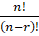Four digit numbers that can be formed with the digits 2, 3, 4, 7 and using each digit only once

=4P4 = 4! = 24

Hence, Option (C) 24 is the correct answer.

29. The sum of the digits in unit place of all the numbers formed with the help of 3, 4, 5 and 6 taken all at a time is
A. 432
B. 108
C. 36
D. 18

Solution:

B. 108

Explanation:

The sum of the digits in unit place of all the numbers formed with the help of 3, 4, 5 and 6 taken all at a time =(3+4+5+6)3! =108

Hence, Option (B) 108 is the correct answer.

30. Total number of words formed by 2 vowels and 3 consonants taken from 4 vowels and 5 consonants is equal to
A. 60
B. 120
C. 7200
D. 720

Solution:

C. 7200

Explanation:

We know that,

nCrTotal number of given vowels=4

Total number of given consonant=5

Total number of words that can be formed by 2 vowels and 3 consonants =4C2.5C3 = 4!/2!2!

2 vowel and 3 consonant =5!

Total number of word = 5! ×4!/2!2! = 7200

Hence, Option (C) 7200 is the correct answer.

31. A five digit number divisible by 3 is to be formed using the numbers 0, 1, 2, 3, 4 and 5 without repetitions. The total number of ways this can be done is
A. 216
B. 600
C. 240
D. 3125
[Hint:5 digit numbers can be formed using digits 0, 1, 2, 4, 5 or by using digits 1, 2, 3, 4, 5 since sum of digits in these cases is divisible by 3.]

Solution:

A. 216

Explanation:

5-digit numbers that can be formed using digits 0, 1, 2, 4, 5

 4 4 3 2 1

4×4×3×2×1=96

5-digit numbers can be formed using digits 1, 2, 3, 4, 5 = 5!

Total number of ways = 5! +96

=216

Hence, Option (A) 216 is the correct answer.

32. Everybody in a room shakes hands with everybody else. The total number of hand shakes is 66. The total number of persons in the room is
A. 11

B. 12
C. 13
D. 14

Solution:

B. 12

Explanation:

We know that,

nCrLet total time of handshakes=nC2 = 66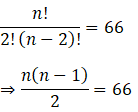⇒ n2-n=132

⇒ (n-12) (n+11) = 0

n=12 or n = – 11

Therefore, n = 12

Hence, Option (B) 12 is the correct answer.